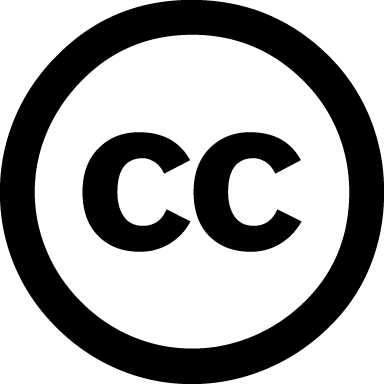https://www.dagstuhl.de/13411

# Deduction and Arithmetic

## Organisatoren

Nikolaj S. Bjørner (Microsoft Corporation – Redmond, US)
Tobias Nipkow (TU München, DE)
Christoph Weidenbach (MPI für Informatik – Saarbrücken, DE)

## Dokumente

Dagstuhl Report, Volume 3, Issue 10Motivationstext
Teilnehmerliste
Dagstuhl's Impact: Dokumente verfügbar
Programm des Dagstuhl-Seminars [pdf]

## Summary

Arithmetic plays a fundamental role in deduction. Logical constraints over arithmetical properties occur frequently in classical theorems in mathematics, as well as in program analysis and verification. The first automatic theorem prover was an implementation of Presburger Arithmetic in 1954. With the availability of powerful predicate calculus proof procedures some years later, arithmetic would be relegated to the sidelines. Interest in arithmetic revived in the 1980s with the advent of powerful interactive theorem provers that needed and supported arithmetic for their applications. The need for efficient computer aided deduction with support for arithmetic in the area of program analysis and verification recently gave birth to a new technology, so called SMT solvers.

Thus we have three strands of automated deduction: SMT solvers, automated first-order provers, and interactive provers in need of (more) arithmetic.

SMT SMT (satisfiability modulo theories) solvers distinguish themselves by integrating built-in support for a combination of theories, including prominently the theory of arithmetic. Most often handling arithmetic formulas in isolation is not sufficient. Applications typically use a non-disjoint combination of arithmetic and other theory reasoning. SMT solvers nowadays handle quantifier-free arithmetic well, but are not directly equipped to solve arithmetical formulas with quantifiers. Recent progress on building in quantifier-elimination procedures for linear and non-linear arithmetic have made practical integration of such richer arithmetic deduction viable.

ATP Research in first-order logic theorem proving used to concentrate on efficient calculi in general and the integration of equational theories in particular. It is obvious that further integration of ``richer'' arithmetic theories into first-order logic should be done by rather a combination approach than an integration approach. One major challenge of combining first-order logic calculi with arithmetic procedures is that of compactness/completeness and termination. While Boolean combinations of ground atoms, as they are considered by SMT solvers typically do not cause trouble with respect to those challenges, combining first-order clauses with an arithmetic theory can never result in a compact/complete/terminating calculus, in general. The actual combination typically requires the solution of purely arithmetic problems in order to establish valid inferences and simplifications. These problems are of a specific nature in that the form of the arithmetic formulas and the way they need to be tested require specific variants of the known arithmetic procedures.

ITP Interactive theorem provers initially came with built-in decision procedures for quantifier-free linear arithmetic. More foundational systems then developed new techniques to implement these decision procedures by reducing them to pure logic, trading efficiency for guaranteed correctness. Aspects of arithmetic reasoning are present in deductive software verification systems: interactive systems combine a number of automatic arithmetic reasoning methods and control them with heuristics that are specific for verification. A challenging application of interactive proof and arithmetic is the Flyspeck project, an effort to formalize Hales's proof of the Kepler conjecture in an interactive theorem prover.

The Dagstuhl seminar was a timely event that brought together experts in the above subareas of deduction, and in reasoning about arithmetic, to exchange experiences and insights. The research questions pursued and answered included:

• Which arithmetic problems are best solved with which approach?
• How to handle very complex numeric representations such as the IEEE floating-point standard with a high degree of automation?
• Arithmetic in combination with other theories results easily in languages with a very complex decision problem---how can a high degree of automation be obtained nevertheless?
• How can SMT-based reasoning be combined with model-based reasoning?
• What is the best way to incorporate arithmetic simplification available in computer algebra systems into deductive frameworks?
• How can the specific structure of arithmetic problems generated by deduction systems be exploited?

In addition to the technical contributions, the seminar participants attempted in an open discussion session to identify the major trends and open questions around Deduction and Arithmetic.Creative Commons BY 3.0 Unported licenseNikolaj S. Bjørner, Reiner Hähnle, Tobias Nipkow, and Christoph Weidenbach

## Classification

• Artificial Intelligence
• Semantics / Formal Methods
• Verification / Logic

## Keywords

• Automated Deduction
• Program Verification
• Arithmetic Constraint Solving

## Dokumentation

In der Reihe Dagstuhl Reports werden alle Dagstuhl-Seminare und Dagstuhl-Perspektiven-Workshops dokumentiert. Die Organisatoren stellen zusammen mit dem Collector des Seminars einen Bericht zusammen, der die Beiträge der Autoren zusammenfasst und um eine Zusammenfassung ergänzt.

## Dagstuhl's Impact

Bitte informieren Sie uns, wenn eine Veröffentlichung ausgehend von Ihrem Seminar entsteht. Derartige Veröffentlichungen werden von uns in der Rubrik Dagstuhl's Impact separat aufgelistet  und im Erdgeschoss der Bibliothek präsentiert.

## Publikationen

Es besteht weiterhin die Möglichkeit, eine umfassende Kollektion begutachteter Arbeiten in der Reihe Dagstuhl Follow-Ups zu publizieren.